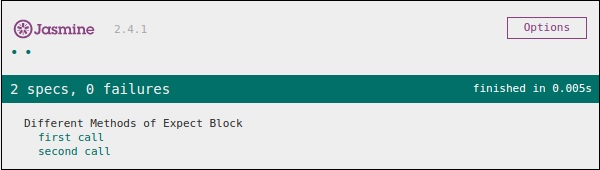# JasmineJS - afterEach()

Like beforeEach(), afterEach() works exactly the same way. It executes after the execution of the spec block. Let us modify the previous example using the following code.

```var currentVal = 0;

afterEach(function() {
currentVal = 5;
});

describe("Different Methods of Expect Block",function() {
it("first call ", function() {
expect(currentVal).toEqual(0);
});

it("second call ",  function() {
expect(currentVal).toEqual(5);
});
});
```

In the above example, while running the first spec block the value of the currentVal is 0. Hence, it will pass the test case but after running the first it block, Jasmine compile ran the afterEach() block, which makes the value of the currentVal to 5. Hence it also satisfies the second case and yields a green screenshot as an output.